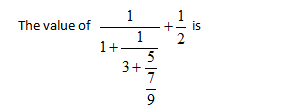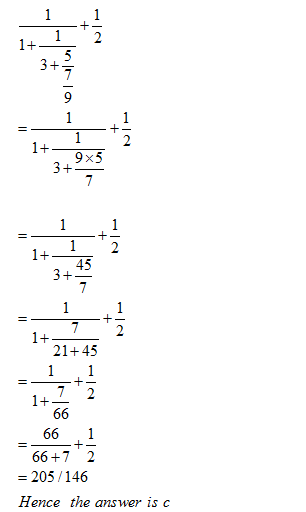• This is an assessment test.
• These tests focus on the basics of Maths and are meant to indicate your preparation level for the subject.
• Kindly take the tests in this series with a pre-defined schedule.

## Basic Maths: Test 3

Congratulations - you have completed Basic Maths: Test 3. You scored %%SCORE%% out of %%TOTAL%%. You correct answer percentage: %%PERCENTAGE%% . Your performance has been rated as %%RATING%%
 Question 1A $\displaystyle \frac{123}{122}$ B $\displaystyle \frac{145}{154}$ C $\frac{205}{146}$ D $\displaystyle \frac{147}{157}$
Question 1 Explanation:
We do solve the first half and then we solve the whole questionQuestion 2
If $\displaystyle x=1+\frac{1}{2+\frac{1}{3+\frac{1}{4+\frac{1}{2}}}}$ Then, the value of $\displaystyle 2x+\frac{1}{2}$ is
 A $\displaystyle 3\frac{49}{134}$ B $\displaystyle 5\frac{13}{24}$ C $\displaystyle 7\frac{13}{24}$ D $\displaystyle 6\frac{23}{134}$
Question 2 Explanation:
In this we need the value of x so for value of x we will solve the complex fraction part first .
$\displaystyle \begin{array}{l}x=1+\frac{1}{2+\frac{1}{3+\frac{1}{4+\frac{1}{2}}}}\\x=1+\frac{1}{2+\frac{1}{3+\frac{2}{9}}}\\x=1+\frac{1}{2+\frac{9}{29}}\\x=1+\frac{29}{67}\\x=96/67\\Now\,by\,\,filling\,\,the\,\,value\,of\,\,x\\we\,\,have\,\,\\2x+\frac{1}{2}=2\times 96/67+\frac{1}{2}\\=\,451/134\,\,=\,\,3\frac{49}{134}\end{array}$
 Question 3
$\displaystyle 16\times 54\div 18=a$
 A 50 B 52 C 44 D None
Question 3 Explanation:
We can simplified the equation as
$\displaystyle a=16\times \frac{54}{18}$ So the value of a will be 48 hence d is the right answer
 Question 4
Find the value of m in $\displaystyle 785-m+12\times 11=6295$
 A -5883 B -5793 C -5378 D -5563
Question 4 Explanation:
The equation can be simplified to
$\displaystyle \begin{array}{l}785-m+12\times 11=6295\\or,\,\,6295+m=785+12\times 11\\m=785+12\times 11-6295\\m=\text{-5378}\end{array}$
Therefore the right answer is option c
 Question 5
If
$\displaystyle {{9}^{5.5}}\times {{9}^{y}}={{9}^{9}}$
then find the value of y
 A 4.5 B 2.29 C 2.75 D none
Question 5 Explanation:
In case of multiplication if bases are same then the power can be added
So here 5.5 + y =9
From here y = 9 - 5.5 = 3.5
Hence the option d is the right answer
Once you are finished, click the button below. Any items you have not completed will be marked incorrect.
There are 5 questions to complete.
 ← List →